### IMO Shortlist 2013 problem N5

Kvaliteta:
Avg: 3,0
Težina:
Avg: 8,0
Fix an integer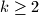$k \geq 2$. Two players, called Ana and Banana, play the following game of numbers. Initially, some integer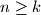$n \geq k$ gets written on the blackboard. Then they make moves in turn, with Ana beginning. A player making a move erases the number$m$ just written on the blackboard and replaced it by some number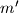$m'$ with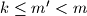$k \leq m' < m$ that is coprime to$m$. The first player who cannot move anymore loses.

An integer$n \geq k$ is called good if Banana has a winning strategy when the initial number is$n$, and bad otherwise.

Consider two integers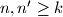$n, n' \geq k$ with the property that each prime number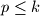$p \leq k$ divides$n$ if and only if it divides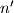$n'$. Prove that either both$n$ and$n'$ are good or both are bad.
Izvor: Italy